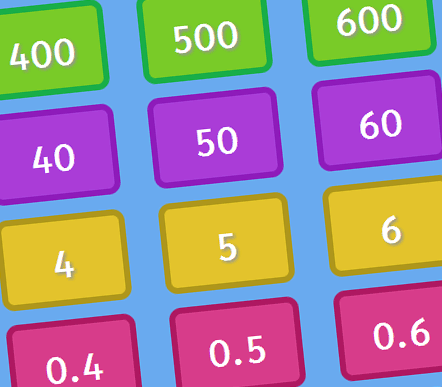# Place Value Charts• Practise
• Teaching Mode

• T O
• H T O
• Th H T O
• T O . t
• T O . t h

• T O
• H T O
• Th H T O
• T O . t
• T O . t h

## Demonstrate how to make a number

### Ideal for Interactive Whiteboards

• T O
• H T O
• Th H T O
• T O . t
• T O . t h
• H T O . t h
• H T O . t h th